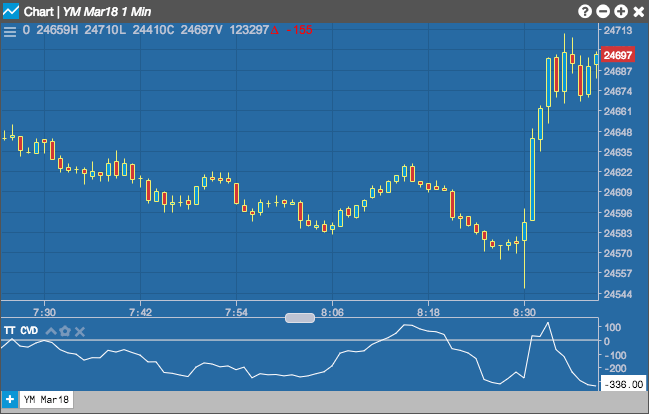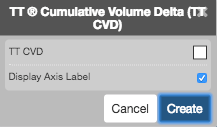Charts

# TT Cumulative Volume Delta (TT_CVD)

The TT Cumulative Volume Delta (TT CVD Study) displays a running total of net transactions as calculated by Volume Delta. Transactions occurring on the Ask are considered Buying Pressure and are added to the total, and those occurring on the Bid are considered Selling Pressure and are subtracted from the cumulative total.## Configuration Options• Color Selectors: Colors to use for graph elements.
• Display Axis Label: Whether to display the most recent value on the Y axis.

## Formula

TT CVD = Cumulative ( Vol Δ )

Vol Δ = Difference between Bid Volume and Ask volume over specified interval

Bid Volume = - ( Accumulated Bid Volume )